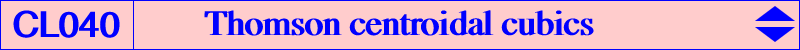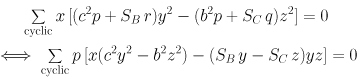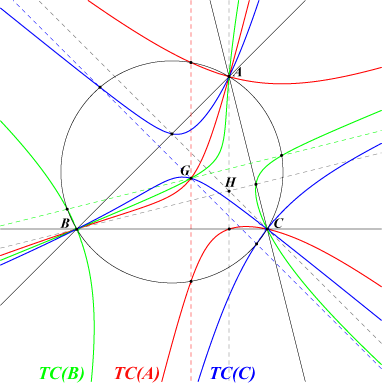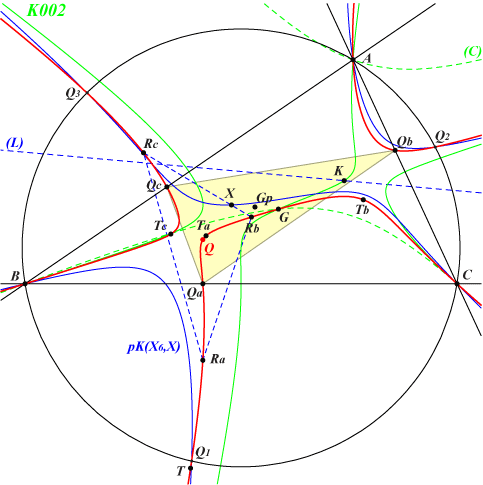Let Q = p:q:r be a fixed point and M a variable point. The locus of M such that the trilinear polar of M is perpendicular to the line QM is a circumcubic TC(Q) passing through G, Q, the vertices Qa, Qb, Qc of the pedal triangle of Q and the points at infinity of the Thomson cubic. TC(Q) is also the locus of the common points of a line through Q with the circumconic passing through G and the trilinear pole of the perpendicular at Q to this line. This gives an easy construction of TC(Q). TC(Q) has equation :All these cubics form a net of K0 cubics i.e. the equation has no term in xyz. This net is generated by the three nodal cubics TC(A), TC(B) and TC(C). TC(A) has node A with two nodal tangents which are the bisectors at A. It contains the foot of the altitude AH and the two common points of the circumcircle and the perpendicular at G to BC. Obviously, TC(A) contains G and the points at infinity of the Thomson cubic K002. *** TC(Q) meets K002 at six finite points that lie on the circum-conic (C) passing through G, whose perspector is the infinite point of any perpendicular to the line OQ when Q ≠ O. When Q = O, TC(Q) is the Thomson cubic itself.TC(Q) meets the circumcircle at A, B, C and three other points Q1, Q2, Q3 which also lie on pK(X6, X) where X is the point with first barycentric coordinate : a^2[3b^2c^2p+c^2q(a^2-c^2)+b^2r(a^2-b^2] X is the reflection of G about the centroid Gp of QaQbQc. X lies on the parallel at Q to the polar line of Q in the Stammler hyperbola. TC(Q) and pK(X6, X) meet again at three points on the line (L), passing through X(6), whose trilinear pole is the isogonal conjugate of the infinite point of any perpendicular to the line OQ when Q ≠ O. TC(Q) also contains Ra = AG /\ QQa and Rb, Rc defined similarly. TC(Q) meets the cevian lines of Q again at Ta, Tb, Tc which are the vertices of a triangle perspective at T to QaQbQc. T lies on TC(Q). Note that G, Q, T are collinear when Q lies on Q012.Pivotal TC(Q) TC(Q) is a pK if and only if QaQbQc (pedal triangle of Q) is a cevian triangle i.e. if and only if Q lies on the Darboux cubic. In this case, the pole of TC(Q) is W, perspector of QaQbQc and anticevian triangle of Q, a point on the Thomson cubic, the pivot of TC(Q) is P, perspector of QaQbQc and ABC, a point on the Lucas cubic. The following table gives a selection of such pivotal TC(Q).Q W P cubic or X(i) on the cubic X(1) X(57) X(7) K365 X(3) X(6) X(2) K002 Thomson cubic X(4) X(4) X(4) K181 X(20) X(2) X(69) K007 Lucas cubic X(40) X(1) X(8) K308 X(64) X(1073) X(253) X(2), X(3), X(64), X(69), X(253), X(1073) X(84) X(282) X(189) X(2), X(8), X(9), X(84), X(189), X(282) X(1490) X(9) X(329) X(2), X(9), X(188), X(282), X(329), X(1034), X(1490) X(1498) X(3) X(20) X(2), X(3), X(20), X(1032), X(1073), X(1498) X(3183) X(1249) X(14361) X(2), X(1249) X(3346) X(3344) X(1032) X(2), X(20), X(1032), X(1249) X(3345) X(3342) X(1034) X(2), X(223), X(329), X(1034) X(3348) X(3349) X(14365) X(2), X(14365)Non-pivotal TC(Q) TC(Q) is a nK (in fact a nK0) if and only if Qa, Qb, Qc are collinear i.e. either : Q lies at infinity in which case TC(Q) decomposes into the line at infinity and a circumconic through G (the perspector is the infinite point of the direction orthogonal to Q), Q lies on the circumcircle. In this case, the pole W of the nK0 is Q and its root P is the trilinear pole of the Simson line of Q which is the line QaQbQc. Hence, P lies on the Simson cubic K010. TC(Q) meets the circumcircle at Q and two other points lying on the parallel at G to the Simson line of Q. The following table gives a selection of such non-pivotal TC(Q).Q = W cubic or X(i) on the cubic X(110) X(2), X(110), X(1113), X(1114) X(691) X(2), X(98), X(110), X(671), X(691) X(1381) X(1), X(2), X(57), X(1381) X(1382) X(1), X(2), X(57), X(1382) X(2709) X(2), X(99), X(111), X(2709) X(2742) X(2), X(100), X(105), X(2742) X(2764) X(2), X(107), X(1297), X(2764)TC(Q) with concurring asymptotes TC(Q) is a K0+ if and only if Q lies on the line X(6)-X(376). The asymptotes concur at a point on the line GK. All these cubics form a pencil which contains two non-degenerated K0++ : K314 = TC(X6) and K315 = TC(X376). These two cubics are central cubics with centers X(6), X(2) respectively.   Singular TC(Q) TC(Q) is singular if and only if Q lies at infinity (in which case it decomposes) or on a 10th degree curve passing through A, B, C, G, X(182), X(390). TC(G) = K295 has a double point at G, TC(X182) = K281 at K, TC(X390) at X(7).   TC(Q) through one or two given point(s) Let S be a given finite point distinct of G. TC(Q) passes through S if and only if Q lies on the perpendicular at S to the trilinear polar of S. Consequently, TC(Q) passes through two given finite points distinct of G if and only if Q is the intersection of two such perpendiculars. For example, for any Q on the Euler line TC(Q) contains H and for any Q on the Brocard line TC(Q) contains K hence TC(Q) contains H and K if and only if Q = O and then TC(Q) is the Thomson cubic.